GeeksforGeeks App
Open AppBrowser
Continue

# Opening Modes in Standard I/O in C/C++ with Examples

Pre-requisites: File Handling in C++

So far the operations using the C program are done on a prompt/terminal that is not stored anywhere. But in the software industry, most of the programs are written to store the information fetched from the program. One such way is to store the fetched information in a file. Different operations that can be performed on a file are:

1. Creation of a new file (fopen with attributes as “a” or “a+” or “w” or “w++”).
2. Opening an existing file (fopen).
3. Reading from file (fscanf or fgets).
4. Writing to a file (fprintf or fputs).
5. Moving to a specific location in a file (fseek, rewind).
6. Closing a file (fclose).

The text in the brackets denotes the functions used for performing those operations.

Why files are needed?

• Data Preservation: Storing data in files helps to preserve data even if the program terminates.
• Easy Data Access: Accessing data becomes easy when there is a large amount of data and it is stored in the file, then this data can be accessed using the C commands.
• Portability: It becomes easier to move data from one computer to another without any changes.

Types of files

They are two types of files that everyone should be aware of-

1. Text Files: Text files are the normal files that have an extension “.txt” in the file name. These can be simply created using editors like Notepad. Upon opening the files the text will be visible like simple plain text and the content can be easily edited or deleted. These are the lowest maintenance files, easy to read. But there are a few disadvantages of text files like they are the least secure files and take bigger storage space.
2. Binary Files: Binary files are “.bin” extension files. Data in these files are stored in the binary form i.e. 0’s and 1’s. These files can hold a large amount of data and provide a higher level of security than text files but these files are not easily readable.

File Operations

There are four basic operations that can be performed on a file:

1. Creating a new file.
2. Opening an existing file.
3. Reading from or writing information to the file.
4. Closing the file.

Working with files

When working with the files, there is a need to declare a pointer of the type file. This file-type pointer is needed for communication between the file and the program.

File *fptr;

Opening a file

Opening a file is done using the fopen() function in the header file stdio.h.

Syntax:

fptr = fopen(“file_name”, “mode”);

Example:

fopen(“D:\\geeksforgeeks\\newprogramgfg.txt”, “w”); fopen(“D:\\geeksforgeeks\\oldprogramgfg.bin”, “rb”);

• Suppose the file newprogramgfg.txt doesn’t exist in the location D:\geeksforgeeks. The first function creates a new file named newprogramgfg.txt and opens it for writing as per the mode ‘w’. The writing mode allows you to create and edit (overwrite) the contents of the file.
• Suppose the second binary file oldprogramgfg.bin exists in the location D:\geeksforgeeks. The second function opens the existing file for reading in binary mode ‘rb’. The reading mode only allows one to read the file, one cannot write into the file.

File Opening modes in C:

Closing a file

The file should be closed after reading or writing. Closing a file is performed using the fclose() function.

Syntax:

fclose(fptr);

Here, fptr is the file type pointer associated with the file to be closed.

Reading and writing to a text file

For reading and writing to a text file, the functions fprintf() and fscanf() are used. They are the file versions of the printf() and scanf() functions. The only difference is that the fprintf() and fscanf() expect the pointer to the structure file.

Write to a text file:

Syntax:

FILE *filePointer; filePointer = fopen(“filename.txt”, “w”)

Below is the C program to write to a text file.

## C

 `// C program to implement``// the above approach``#include ``#include `` ` `// Driver code``int` `main()``{``    ``// Declare the file pointer``    ``FILE``* filePointer;`` ` `    ``// Get the data to be written in file``    ``char` `dataToBeWritten``        ``= ``"GeeksforGeeks-A Computer"``          ``+ ``" Science Portal for Geeks"``;`` ` `    ``// Open the existing file GfgTest.c using fopen()``    ``// in write mode using "w" attribute``    ``filePointer = ``fopen``(``"GfgTest.txt"``, ``"w"``);`` ` `    ``// Check if this filePointer is null``    ``// which maybe if the file does not exist``    ``if` `(filePointer == NULL) {``        ``printf``(``"GfgTest.txt file failed to open."``);``    ``}``    ``else` `{``        ``printf``(``"The file is now opened.\n"``);`` ` `        ``// Write the dataToBeWritten into the file``        ``if` `(``strlen``(dataToBeWritten) > 0) {``            ``// writing in the file using fputs()``            ``fprintf``(filePointer, dataToBeWritten);``            ``fprintf``(filePointer, ``"\n"``);``        ``}`` ` `        ``// Closing the file using fclose()``        ``fclose``(filePointer);`` ` `        ``printf``(``"Data successfully written"``               ``+ ``" in file GfgTest.txt\n"``);``        ``printf``(``"The file is now closed."``);``    ``}``    ``return` `0;``}`

Output: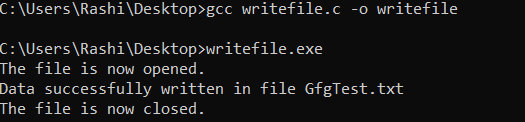Syntax:

FILE * filePointer; filePointer = fopen(“fileName.txt”, “r”);

Below is the C program to read text file.

## C

 `// C program to implement``// the above approach``#include ``#include `` ` `// Driver code``int` `main()``{``    ``// Declare the file pointer``    ``FILE``* filePointer;`` ` `    ``// Declare the variable for the data``    ``// to be read from file``    ``char` `dataToBeRead;`` ` `    ``// Open the existing file GfgTest.txt``    ``// using fopen() in read mode using``    ``// "r" attribute``    ``filePointer = ``fopen``(``"GfgTest.txt"``, ``"r"``);`` ` `    ``// Check if this filePointer is null``    ``// which maybe if the file does not exist``    ``if` `(filePointer == NULL) {``        ``printf``(``"GfgTest.txt file failed to open."``);``    ``}``    ``else` `{``        ``printf``(``"The file is now opened.\n"``);`` ` `        ``// Read the dataToBeRead from the file``        ``// using fgets() method``        ``while` `(``fgets``(dataToBeRead, 50,``                     ``filePointer)``               ``!= NULL) {``            ``// Print the dataToBeRead``            ``printf``(``"%s"``, dataToBeRead);``        ``}`` ` `        ``// Closing the file using fclose()``        ``fclose``(filePointer);`` ` `        ``printf``(``"Data successfully read"``               ``+ ``" from file GfgTest.txt\n"``);``        ``printf``(``"The file is now closed."``);``    ``}``    ``return` `0;``}`

Output: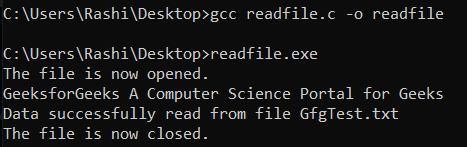Reading and writing in binary file

Write a binary file:

Syntax:

FILE *filePointer; filePointer = fopen(“fileName.bin”, “wb”);

To write data to a binary file, fwrite() function is needed. This function takes four arguments:

1. Address of data to be written in the disk.
2. Size of data to be written on the disk.
3. The number of such types of data.
4. Pointer to the file where you want to write.

Syntax:

Below is the C program to implement the above approach:

## C

 `// C program to implement``// the above approach``#include ``#include `` ` `struct` `threeNum {``    ``int` `n1, n2, n3;``};`` ` `// Driver code``int` `main()``{``    ``int` `n;``    ``struct` `threeNum num;`` ` `    ``// Declaring the file pointer``    ``FILE``* fptr;`` ` `    ``if` `((fptr = ``fopen``(``"C:\\GfgTest.bin"``,``                      ``"wb"``))``        ``== NULL) {``        ``printf``(``"Error! opening file"``);`` ` `        ``// Program exits if the file pointer``        ``// returns NULL.``        ``exit``(1);``    ``}`` ` `    ``for` `(n = 1; n < 5; ++n) {``        ``num.n1 = n;``        ``num.n2 = 5 * n;``        ``num.n3 = 5 * n + 1;``        ``fwrite``(&num, ``sizeof``(``struct` `threeNum),``               ``1, fptr);``    ``}`` ` `    ``printf``(``"The file GfgTest.bin is"``           ``+ ``" written successfully"``);``    ``fclose``(fptr);``    ``return` `0;``}`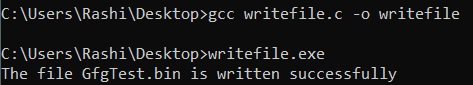Syntax:

FILE * filePointer; filePointer = fopen(“fileName.txt”, “rb”);

To read data from a binary file, fread(0 function is used. Similar to fwrite() function, this function also takes four arguments.

Syntax:

Below is the C program to implement the above approach:

## C

 `// C program to implement``// the above approach``#include ``#include `` ` `struct` `threeNum {``    ``int` `n1, n2, n3;``};`` ` `// Driver code``int` `main()``{``    ``int` `n;``    ``struct` `threeNum num;`` ` `    ``// Declaring the file pointer``    ``FILE``* fptr;`` ` `    ``if` `((fptr = ``fopen``(``"C:\\GfgTest.bin"``,``                      ``"rb"``))``        ``== NULL) {``        ``printf``(``"Error! opening file"``);`` ` `        ``// Program exits if the file pointer``        ``// returns NULL.``        ``exit``(1);``    ``}`` ` `    ``for` `(n = 1; n < 5; ++n) {``        ``fread``(&num, ``sizeof``(``struct` `threeNum),``              ``1, fptr);``        ``printf``(``"n1: %d\tn2: %d\tn3: %d"``,``               ``num.n1, num.n2, num.n3);``        ``printf``(``"\n"``);``    ``}``    ``fclose``(fptr);`` ` `    ``return` `0;``}`

Output: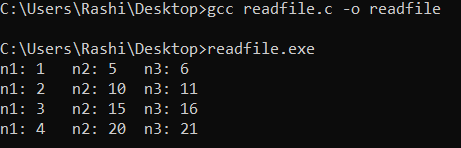Append content in text file

Syntax:

FILE * filePointer; filePointer = fopen(“fileName.txt”, “a”);

Once file is opened in append mode, rest of the task is same as that to write content in a text file.

Below is the example to append a string to the file:

## C

 `// C program to implement``// the above approach``#include ``#include `` ` `// Driver code``int` `main()``{``    ``// Declare the file pointer``    ``FILE``* filePointer;`` ` `    ``// Get the data to be appended in file``    ``char` `dataToBeWritten``        ``= ``"It is a platform for"``          ``+ ``" learning language"``          ``+ ``" tech related topics"``;`` ` `    ``// Open the existing file GfgTest.txt using``    ``// fopen() in append mode using "a" attribute``    ``filePointer = ``fopen``(``"GfgTest.txt"``, ``"a"``);`` ` `    ``// Check if this filePointer is null``    ``// which maybe if the file does not exist``    ``if` `(filePointer == NULL) {``        ``printf``(``"GfgTest.txt file failed to open."``);``    ``}``    ``else` `{``        ``printf``(``"The file is now opened.\n"``);`` ` `        ``// Append the dataToBeWritten into the file``        ``if` `(``strlen``(dataToBeWritten) > 0) {``            ``// writing in the file using fputs()``            ``fprintf``(filePointer, dataToBeWritten);``            ``fprintf``(filePointer, ``"\n"``);``        ``}`` ` `        ``// Closing the file using fclose()``        ``fclose``(filePointer);`` ` `        ``printf``(``"Data successfully appended"``               ``+ ``" in file GfgTest.txt\n"``);``        ``printf``(``"The file is now closed."``);``    ``}``    ``return` `0;``}`

Output: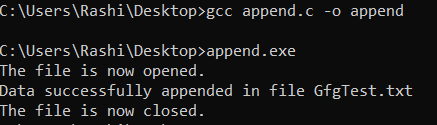Append content in binary file

Syntax:

FILE * filePointer; filePointer = fopen(“fileName.bin”, “ab”);

Once file is opened in append mode, rest of the task is same as that to write content in a binary file.

## C

 `// C program to implement``// the above approach``#include ``#include `` ` `struct` `threeNum {``    ``int` `n1, n2, n3;``};`` ` `// Driver code``int` `main()``{``    ``int` `n;``    ``struct` `threeNum num;`` ` `    ``// Declaring the file pointer``    ``FILE``* fptr;`` ` `    ``// Opening the file in``    ``// append mode``    ``if` `((fptr = ``fopen``(``"C:\\GfgTest.bin"``,``                      ``"ab"``))``        ``== NULL) {``        ``printf``(``"Error! opening file"``);`` ` `        ``// Program exits if the file pointer``        ``// returns NULL.``        ``exit``(1);``    ``}`` ` `    ``for` `(n = 1; n < 10; ++n) {``        ``num.n1 = n;``        ``num.n2 = 5 * n;``        ``num.n3 = 5 * n + 1;``        ``fwrite``(&num, ``sizeof``(``struct` `threeNum),``               ``1, fptr);``    ``}`` ` `    ``printf``(``"The file GfgTest.bin"``           ``+ ``" is appended successfully"``);``    ``fclose``(fptr);``    ``return` `0;``}`

Output:Opening file for both reading and writing

Syntax:

FILE * filePointer; filePointer = fopen(“fileName.txt”, “r+”);

The file is opened using the mode “r+'” and the file is opened in both reading and writing mode.

## C

 `// C program to implement``// the above approach``#include ``#include `` ` `// Driver code``int` `main()``{``    ``// Declare the file pointer``    ``FILE``* filePointer;``    ``char` `dataToBeWritten``        ``= ``"It is a platform for"``          ``+ ``" learning language"``          ``+ ``" tech related topics."``;`` ` `    ``// Declare the variable for the data``    ``// to be read from file``    ``char` `dataToBeRead;`` ` `    ``// Open the existing file GfgTest.txt``    ``// using fopen() in read mode using``    ``// "r+" attribute``    ``filePointer = ``fopen``(``"GfgTest.txt"``, ``"r+"``);`` ` `    ``// Check if this filePointer is null``    ``// which maybe if the file does not exist``    ``if` `(filePointer == NULL) {``        ``printf``(``"GfgTest.txt file failed to open."``);``    ``}``    ``else` `{``        ``printf``(``"The file is now opened.\n"``);`` ` `        ``// Read the dataToBeRead from the file``        ``// using fgets() method``        ``while` `(``fgets``(dataToBeRead, 50,``                     ``filePointer)``               ``!= NULL) {``            ``// Print the dataToBeRead``            ``printf``(``"%s"``, dataToBeRead);``        ``}``        ``printf``(``            ``"\nData successfully read"``            ``+ ``" from file GfgTest.txt"``);`` ` `        ``if` `(``strlen``(dataToBeWritten) > 0) {``            ``// writing in the file using fprintf()``            ``fprintf``(filePointer, dataToBeWritten);``            ``fprintf``(filePointer, ``"\n"``);``        ``}`` ` `        ``printf``(``"\nData successfully"``               ``+ ``" written to the file"``);`` ` `        ``// Closing the file using fclose()``        ``fclose``(filePointer);`` ` `        ``printf``(``"\nThe file is now closed."``);``    ``}``    ``return` `0;``}`

Output: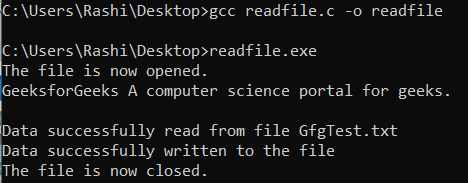Opening file for both reading and writing in binary mode

Syntax:

FILE * filePointer; filePointer = fopen(“fileName.bin”, “rb+”);

## C

 `// C program to implement``// the above approach``#include ``#include `` ` `struct` `threeNum {``    ``int` `n1, n2, n3;``};`` ` `// Driver code``int` `main()``{``    ``int` `n;``    ``struct` `threeNum num;`` ` `    ``// Declaring the file pointer``    ``FILE``* fptr;`` ` `    ``if` `((fptr = ``fopen``(``"C:\\GfgTest.bin"``,``                      ``"rb"``))``        ``== NULL) {``        ``printf``(``"Error! opening file"``);`` ` `        ``// Program exits if the file pointer``        ``// returns NULL.``        ``exit``(1);``    ``}`` ` `    ``for` `(n = 1; n < 5; ++n) {``        ``fread``(&num, ``sizeof``(``struct` `threeNum),``              ``1, fptr);``        ``printf``(``"n1: %d\tn2: %d\tn3: %d"``,``               ``num.n1, num.n2, num.n3);``        ``printf``(``"\n"``);``    ``}``    ``printf``(``"Data successfully read from the file"``);`` ` `    ``for` `(n = 1; n < 7; ++n) {``        ``num.n1 = n;``        ``num.n2 = 5 * n;``        ``num.n3 = 5 * n + 1;``        ``fwrite``(&num, ``sizeof``(``struct` `threeNum),``               ``1, fptr);``    ``}`` ` `    ``printf``(``"The file GfgTest.bin"``           ``+ ``" is written successfully"``);``    ``fclose``(fptr);`` ` `    ``return` `0;``}`

Output: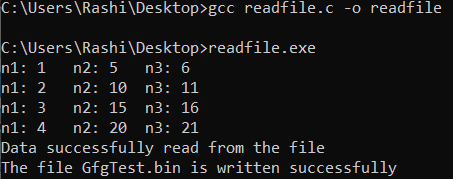Opening file for both reading and writing in text mode

In this mode, the file is opened for both reading and writing in text mode. If the file exists, then the content is overwritten in the file, and in case the file does not exist then in that case, a new file is created.

Syntax:

FILE * filePointer; filePointer = fopen(“fileName.txt”, “w+”);

## C

 `// C program to implement``// the above approach``#include ``#include `` ` `// Driver code``int` `main()``{``    ``// Declare the file pointer``    ``FILE``* filePointer;``    ``char` `dataToBeWritten``        ``= ``"It is a platform"``          ``+ ``" for learning language"``          ``+ ``" tech related topics."``;`` ` `    ``// Declare the variable for the data``    ``// to be read from file``    ``char` `dataToBeRead;`` ` `    ``// Open the existing file GfgTest.txt``    ``// using fopen() in read mode using``    ``// "r+" attribute``    ``filePointer = ``fopen``(``"GfgTest.txt"``, ``"w+"``);`` ` `    ``// Check if this filePointer is null``    ``// which maybe if the file does not exist``    ``if` `(filePointer == NULL) {``        ``printf``(``"GfgTest.txt file failed to open."``);``    ``}``    ``else` `{``        ``printf``(``"The file is now opened.\n"``);`` ` `        ``if` `(``strlen``(dataToBeWritten) > 0) {``            ``// writing in the file using fprintf()``            ``fprintf``(filePointer, dataToBeWritten);``            ``fprintf``(filePointer, ``"\n"``);``        ``}`` ` `        ``printf``(``"Data successfully"``               ``+ ``" written to the file\n"``);`` ` `        ``// Read the dataToBeRead from the file``        ``// using fgets() method``        ``while` `(``fgets``(dataToBeRead, 50,``                     ``filePointer)``               ``!= NULL) {``            ``// Print the dataToBeRead``            ``printf``(``"%s"``, dataToBeRead);``        ``}``        ``printf``(``"\nData successfully read"``               ``+ ``" from file GfgTest.txt"``);`` ` `        ``// Closing the file using fclose()``        ``fclose``(filePointer);`` ` `        ``printf``(``"\nThe file is now closed."``);``    ``}``    ``return` `0;``}`

My Personal Notes arrow_drop_up